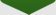\$height) { \$percentage = (\$target / \$width); } else { \$percentage = (\$target / \$height); } //gets the new value and applies the percentage, then rounds the value \$width = round(\$width * \$percentage); \$height = round(\$height * \$percentage); //returns the new sizes in html image tag format...this is so you //can plug this function inside an image tag and just get the return "width=\"\$width\" height=\"\$height\""; } ?> <?php echo \$row_getblog['name']; ?> Blog, By <?php echo \$row_getblog['writer']; ?> InsideTheU.comBy:

Currently no Post, come back soon250 ) { ?>border="0" align="left" hspace="5" >